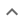•# Simplifying square roots

We think you wrote:

###This solution deals with simplifying square roots.

Solution found16a^6b^10

## Step by Step Solution## Step  1  :

#### Simplify the Integer part of the SQRT

Factor 256 into its prime factors
256 = 28

To simplify a square root, we extract factors which are squares, i.e., factors that are raised to an even exponent.

Factors which will be extracted are :
256 = 28

No factors remain inside the root !!

To complete this part of the simplification we take the squre root of the factors which are to be extracted. We do this by dividing their exponents by 2 :
16 = 24

At the end of this step the partly simplified SQRT looks like this:
16 sqrt(a12b20)

## Step  2  :

#### Simplify the Variable part of the SQRT

Rules for simplifing variables which may be raised to a power:

(1) variables with no exponent stay inside the radical
(2) variables raised to power 1 or (-1) stay inside the radical
(3) variables raised to an even exponent: Half the exponent taken out, nothing remains inside the radical. examples:
(3.1) sqrt(x8)=x4
(3.2) sqrt(x-6)=x-3

(4) variables raised to an odd exponent which is  >2  or  <(-2) , examples:
(4.1) sqrt(x5)=x2•sqrt(x)
(4.2) sqrt(x-7)=x-3•sqrt(x-1)

Applying these rules to our case we find out that

SQRT(a12b20) = a6b10

#### Combine both simplifications

sqrt (256a12b20) =
16 a6b10

16 a6b10